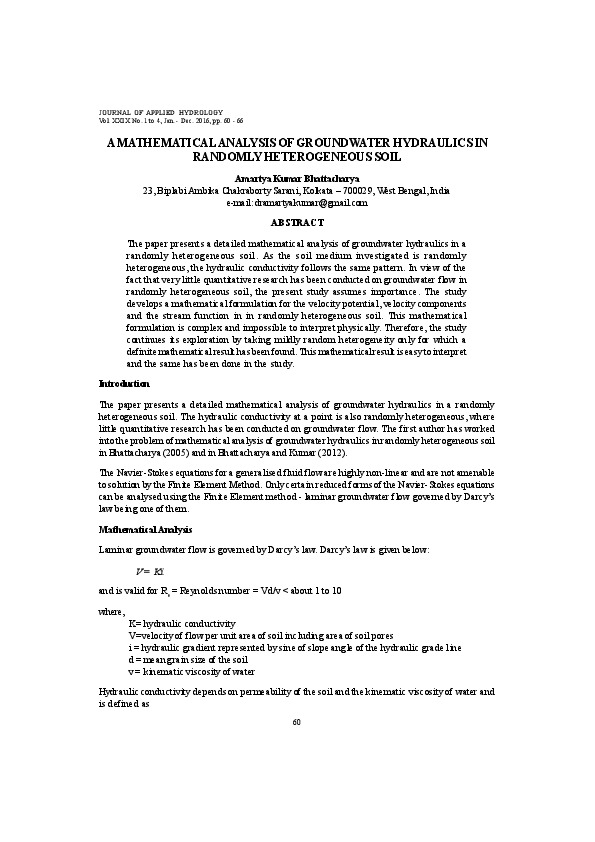# A Mathematical Analysis of Groundwater Hydraulics in Randomly Heterogeneous SoilAbstract

The paper presents a detailed mathematical analysis of groundwater hydraulics in randomly heterogeneous soil. As the soil medium investigated is randomly heterogeneous, the hydraulic conductivity follows the same pattern. In view of the fact that very little quantitative research has been conducted on groundwater flow in randomly heterogeneous soil, the present study assumes importance.

The study develops a mathematical formulation for the velocity potential, velocity components and the stream function in in randomly heterogeneous soil. This mathematical formulation is complex and impossible to interpret physically. Therefore, the study continues its exploration by taking mildly random heterogeneity only for which a definite mathematical result has been found. This mathematical result is easy to interpret and the same has been done in the study.

Find the study attached below.